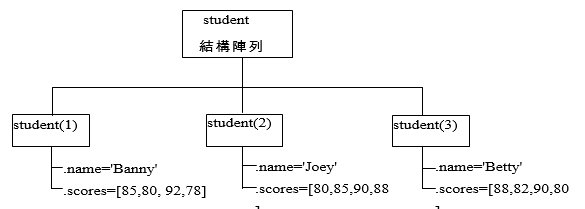13-1 嚙踝蕭嚙踝蕭嚙踝蕭嚙踝蕭嚙踝蕭嚙踝蕭嚙踝蕭嚙踝蕭嚙踝蕭嚙踝蕭嚙?

Example 1: 13-結構陣列/struct01.mclear student % 清除 student 變數 student.name = '洪鵬翔'; % 加入 name 欄位 student.id = 'mr871912'; % 加入 id 欄位 student.scores = [58, 75, 62]; % 加入 scores 欄位 student % 秀出結果 student = name: '洪鵬翔' id: 'mr871912' scores: [58 75 62]

Example 2: 13-結構陣列/struct02.mclear student % 清除 student 變數 student.name = '洪鵬翔'; % 加入 name 欄位 student.id = 'mr871912'; % 加入 id 欄位 student.scores = [58, 75, 62]; % 加入 scores 欄位 % 以下是新加入的第二筆資料 student(2).name = '邱中人'; student(2).id = 'mr872510'; student(2).scores = [25, 36, 92]; student % 秀出結果 student = 1x2 <a href="matlab:helpPopup struct" style="font-weight:bold">struct</a> array with fields: name id scores

student(1)'洪鵬翔''mr871912'[58,75,62]
student(2)'邱中人''mr872510'[25,36,92]

structureArray = struct(field1, value1, field2, value2,….)

Example 3: 13-結構陣列/struct03.mstudent = struct('name', {'張庭碩', '張庭安'}, 'scores', {[50 60], [60 70]}); student(1) % 顯示 student(1) student(2) % 顯示 student(2) ans = name: '張庭碩' scores: [50 60] ans = name: '張庭安' scores: [60 70]

Example 4: 13-結構陣列/struct04.mstudent = struct('name', '張庭安', 'scores', {[50 60], [90 100]}); student(1) % 顯示 student(1) student(2) % 顯示 student(2) ans = name: '張庭安' scores: [50 60] ans = name: '張庭安' scores: [90 100]

Example 5: 13-結構陣列/struct05.mstudent = struct('name', {'張庭碩', '張庭安'}, 'scores', {[50 60], [60 70]}); student(2).course(1).title = 'Web Programming'; student(2).course(1).credits = 2; student(2).course(2).title = 'Numerical Method'; student(2).course(2).credits = 3; student(2).course ans = 1x2 <a href="matlab:helpPopup struct" style="font-weight:bold">struct</a> array with fields: title credits

Hint

Example 6: 13-結構陣列/buildStruct01.mclear student % 清除 student 變數 student(1) = struct('name', 'Banny', 'scores', [85,80,92,78]); student(2) = struct('name', 'Joey', 'scores', [80,85,90,88]); student(3) = struct('name', 'Betty', 'scores', [88,82,90,80]);MATLAB程式設計：入門篇